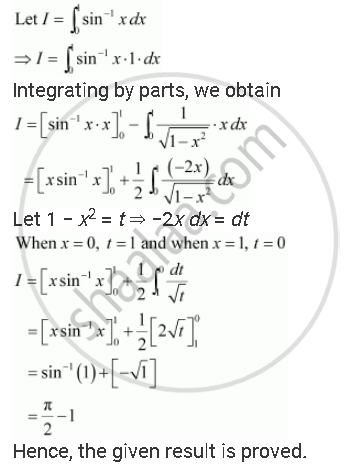Share

# Prove the Following Int_0^1sin^(-1) Xdx = Pi/2 - 1 - Mathematics

#### Question

Prove the following int_0^1sin^(-1) xdx = pi/2 - 1

#### SolutionIs there an error in this question or solution?

#### Video TutorialsVIEW ALL 

Solution Prove the Following Int_0^1sin^(-1) Xdx = Pi/2 - 1 Concept: Definite Integral as the Limit of a Sum.
S